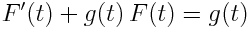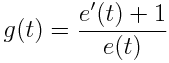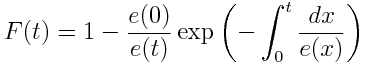# Mean residual time

If something has survived this far, how much longer is it expected to survive? That’s the question answered by mean residual time.

For a positive random variable X, the mean residual time for X is a function eX(t) given byprovided the expectation and integral converge. Here F(t) is the CDF, the probability that X is less than t.

For an exponential distribution, the mean residual time is constant. For a Pareto (power law) distribution, the mean residual time is proportional to t. This has an interesting consequence, known as the Lindy effect.

Now let’s turn things around. Given function a function e(t), can we find a density function for a positive random variable with that mean residual time? Yes.

The equation above yields a differential equation for F, the CDF of the distribution.

If we differentiate both sides ofwith respect to t and rearrange, we get the first order differential equationwhereThe initial condition must be F(0) = 0 because we’re looking for the distribution of a positive random variable, i.e. the probability of X being less than zero must be 0. The solution is thenThis means that for a desired mean residual time, you can use the equation above to create a CDF function to match. The derivative of the CDF function gives the PDF function, so differentiate both sides to get the density.

## 11 thoughts on “Mean residual time”

1. Charlie

Interesting, but could you sketch how you found the solution? My DE is rusty. Thanks!

2. A little confused about your expectation. $$\frac{1}{1-F_x(t)} \int_t^\infty 1-F_x(x) dx$$. Can you comment on why this is true starting from the definition of the expectation in terms of the density? I suspect there is some kind of trick here that would be useful to know.

3. Also could you consider putting a link to an informative page on your banner that instructs in the use of LaTeX on your blog (maybe next to “most popular” you could have “Math notation on this blog”). There seem to be several standard ways to get math in wordpress and the probability of guessing the right one is too small.

4. Charlie: In general, the way to solve an equation of the form y’ + gy = f is to multiply both sides by the integrating factor exp(G) where G’ = g. Then the left side becomes (exp(G) y)’, i.e. a derivative, so you can integrate.

Then exp(G) y = integral of exp(G) f. Then divide by exp(G).

5. Daniel: I don’t have anything installed on my blog to compile LaTeX. The equations above are images produced manually. It’s less convenient, but more robust. You can use HTML in comments. Here are some notes on math in HTML.

E(X – t | X > t) is the integral from t to infinity of (x-t) dF. You can use integration by parts from there to get the integral in the first equation.

6. Good old integration by parts. And really? You do all your math by hand and upload the images :-) you should check out LaTeX for wordpress http://wordpress.org/plugins/latex/ I use it and have found it to be reasonably good and it uses MathJax to render if your browser supports it (most do these days) otherwise it inserts automatically generated images.

7. Charlie

Thanks John!

8. Giles Warrack

“Here F(t) is the CDF, the probability that X is greater than t”

don’t you mean “\le” John?

9. Giles: Yes, thanks.

10. For those who come to this and can’t work through the integration by parts, here’s a proof of the first equality:

http://www.degruyter.com/view/j/tmmp.2012.52.issue-1/v10127-012-0025-9/v10127-012-0025-9.xml

I spent several hours trying to force my way through the integration by parts. It’s not so trivial (in my eyes) to show that the ‘uv’ term has to drop.

Very neat result. Apparently this ‘trick’ is very popular in the actuarial circles (for obvious reasons). But it seems useful enough for any probabilitist / statistician to have in his / her back pocket.

Great post as always, John!